### OPSCORE, MONOTONE, UNTIE, and LINEAR Transformations

Two vectors of information are needed to produce the optimally scaled variable: the initial variable scaling vectorand the target vector. For convenience, both vectors are first sorted on the values of the initial scaling vector. If you request an UNTIE transformation, the target vector is sorted within ties in the initial scaling vector. The normal SAS collating sequence for missing and nonmissing values is used. Sorting simply permits the constraints to be specified in terms of relationships among adjoining coefficients. The sorting process partitionsandinto missing and nonmissing parts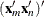, and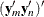.

Next, PROC TRANSREG determines category membership. Every ordinary missing value (.) forms a separate category. (Three ordinary missing values form three categories.) Every special missing value within the range specified in the UNTIE= a-option forms a separate category. (If UNTIE= BC and there are three .B and two .C missing values, five categories are formed from them.) For all other special missing values, a separate category is formed for each different value. (If there are four .A missing values, one category is formed from them.)

Each distinct nonmissing value forms a separate category for OPSCORE and MONOTONE transformations (1 1 1 2 2 3 form three categories). Each nonmissing value forms a separate category for all other transformations (1 1 1 2 2 3 form six categories). When category membership is determined, category means are computed. Here is an example:: (. . .A .A .B 1 1 1 2 2 3 3 3 4)': (5 6 2 4 2 1 2 3 4 6 4 5 6 7)' OPSCORE and MONOTONE means: (5 6 3 2 2 5 5 7)' other means: (5 6 3 2 1 2 3 4 6 4 5 6 7)'

The category means are the coefficients of a category indicator design matrix. The category means are the Fisher (1938) optimal scores. For MONOTONE and UNTIE transformations, order constraints are imposed on the category means for the nonmissing partition by merging categories that are out of order. The algorithm checks upward until an order violation is found, and then averages downward until the order violation is averaged away. (The average of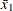computed from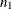observations and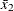computed from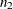observations is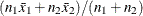.) The MONOTONE algorithm (Kruskal 1964, secondary approach to ties) for this example with means for the nonmissing values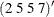would do the following checks: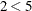: OK,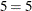: OK,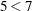: OK. The means are in the proper order, so no work is needed.

The UNTIE transformation (Kruskal 1964, primary approach to ties) uses the same algorithm on the means of the nonmissing values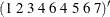but with different results for this example: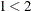: OK,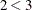: OK,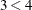: OK,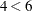: OK,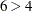: average 6 and 4 and replace 6 and 4 by the average. The new means of the nonmissing values are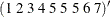. The check resumes: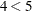: OK,: OK,: OK,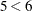: OK,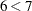: OK. If some of the special missing values are ordered, the upward-checking, downward-averaging algorithm is applied to them also, independently of the other missing and nonmissing partitions. When the means conform to any required category or order constraints, an optimally scaled vector is produced from the means. The following example results from a MONOTONE transformation:: (. . .A .A .B 1 1 1 2 2 3 3 3 4): (5 6 2 4 2 1 2 3 4 6 4 5 6 7)result: (5 6 3 3 2 2 2 2 5 5 5 5 5 7)The upward-checking, downward-averaging algorithm is equivalent to creating a category indicator design matrix, solving for least squares coefficients with order constraints, and then computing the linear combination of design matrix columns.

For the optimal transformation LINEAR and for nonoptimal transformations, missing values are handled as just described. The nonmissing target values are regressed onto the matrix defined by the nonmissing initial scaling values and an intercept. In this example, the target vector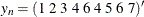is regressed onto the design matrix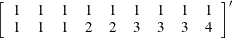Although only a linear transformation is performed, the effect of a linear regression optimal scaling is not eliminated by the later standardization step (unless the variable has no missing values). In the presence of missing values, the linear regression is necessary to minimize squared error.